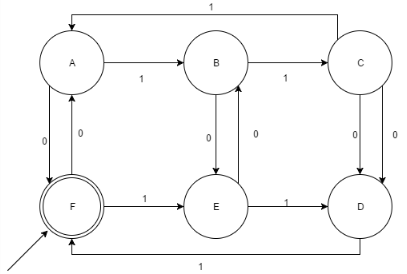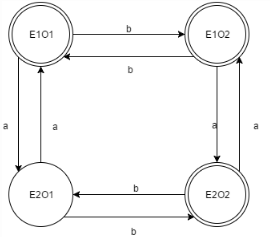Courses

# Test: The Language of DFA

## 10 Questions MCQ Test Theory of Computation | Test: The Language of DFA

Description
This mock test of Test: The Language of DFA for Computer Science Engineering (CSE) helps you for every Computer Science Engineering (CSE) entrance exam. This contains 10 Multiple Choice Questions for Computer Science Engineering (CSE) Test: The Language of DFA (mcq) to study with solutions a complete question bank. The solved questions answers in this Test: The Language of DFA quiz give you a good mix of easy questions and tough questions. Computer Science Engineering (CSE) students definitely take this Test: The Language of DFA exercise for a better result in the exam. You can find other Test: The Language of DFA extra questions, long questions & short questions for Computer Science Engineering (CSE) on EduRev as well by searching above.
QUESTION: 1

### How many languages are over the alphabet R?

Solution:

A language over an alphabet R is a set of strings over A which is uncountable and infinite.

QUESTION: 2

### According to the 5-tuple representation i.e. FA= {Q, ∑, δ, q, F}Statement 1: q ϵ Q’; Statement 2: FϵQ

Solution:

Q is the Finite set of states, whose elements i.e. the states constitute the finite automata.

QUESTION: 3

### δˆ tells us the best:

Solution:

δ or the Transition function describes the best, how a DFA behaves on a string where to transit next, which direction to take.

QUESTION: 4

Which of the following option is correct?A= {{abc, aaba}. {ε, a, bb}}

Solution:

As the question has dot operation, ε will not be a part of the concatenated set. Had it been a union operation, ε would be a part of the operated set.

QUESTION: 5

For a DFA accepting binary numbers whose decimal equivalent is divisible by 4, what are all the possible remainders?

Solution:

All the decimal numbers on division would lead to only 4 remainders i.e. 0,1,2,3 (Property of Decimal division).

QUESTION: 6

Which of the following x is accepted by the given DFA (x is a binary string ∑= {0,1})?Solution:

Explanation : The given DFA accepts all the binary strings such that they are divisible by 3 and 2.Thus, it can be said that it also accepts all the strings which is divisible by 6.

QUESTION: 7

Given:
L1= {xϵ ∑*|x contains even no’s of 0’s}
L2= {xϵ ∑*|x contains odd no’s of 1’s}
No of final states in Language L1 U L2?

Solution:QUESTION: 8

The maximum number of transition which can be performed over a state in a DFA?∑= {a, b, c}

Solution:

The maximum number of transitions which a DFA allows for a language is the number of elements the transitions constitute.

QUESTION: 9

The maximum sum of in degree and out degree over a state in a DFA can be determined as:

∑= {a, b, c, d}

Solution:

The out degree for a DFA I fixed while the in degree depends on the number of states in the DFA and that cannot be determined without the dependence over the Language.

QUESTION: 10

The sum of minimum and maximum number of final states for a DFA n states is equal to:

Solution:

The maximum number of final states for a DFA can be total number of states itself and minimum would always be 1, as no DFA exits without a final state. Therefore, the solution is n+1.